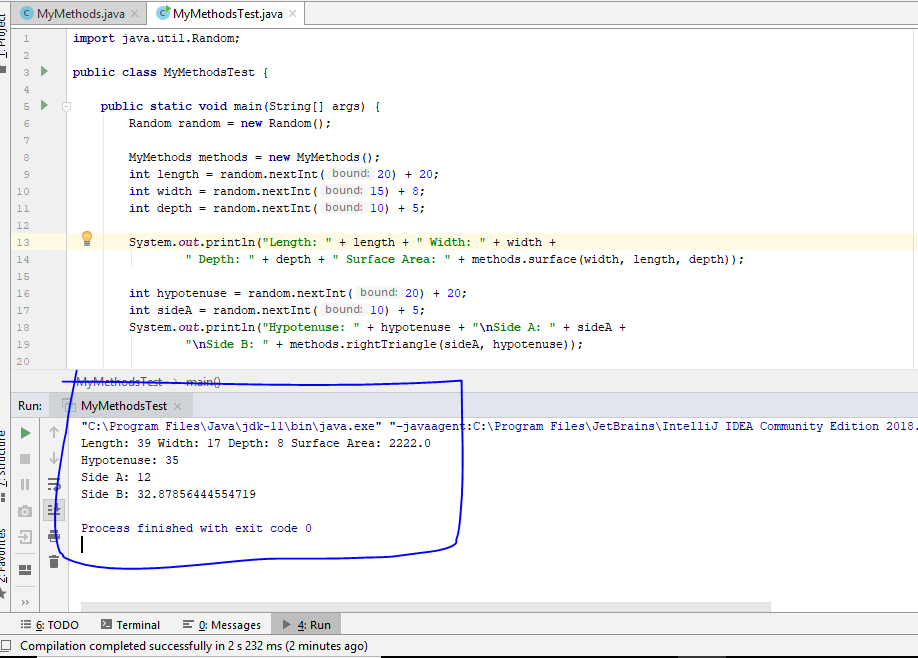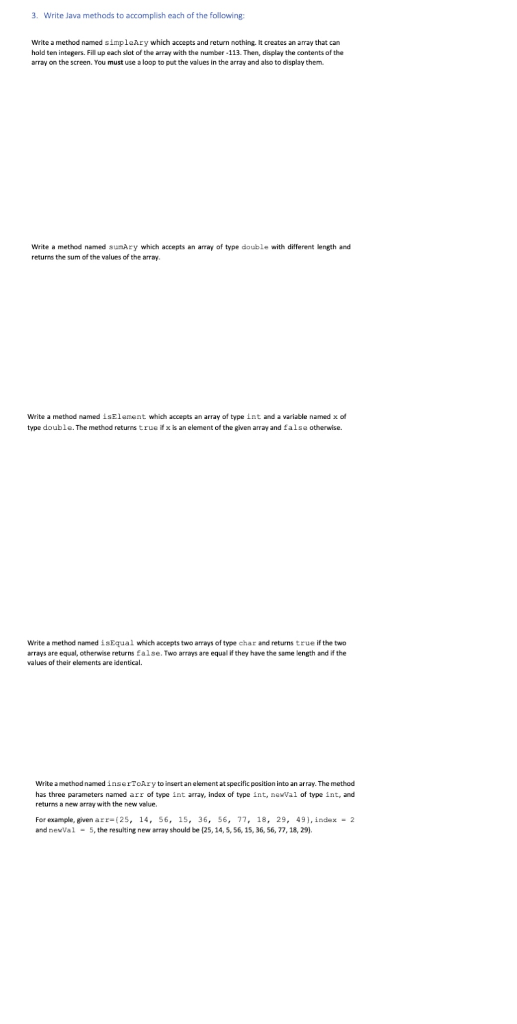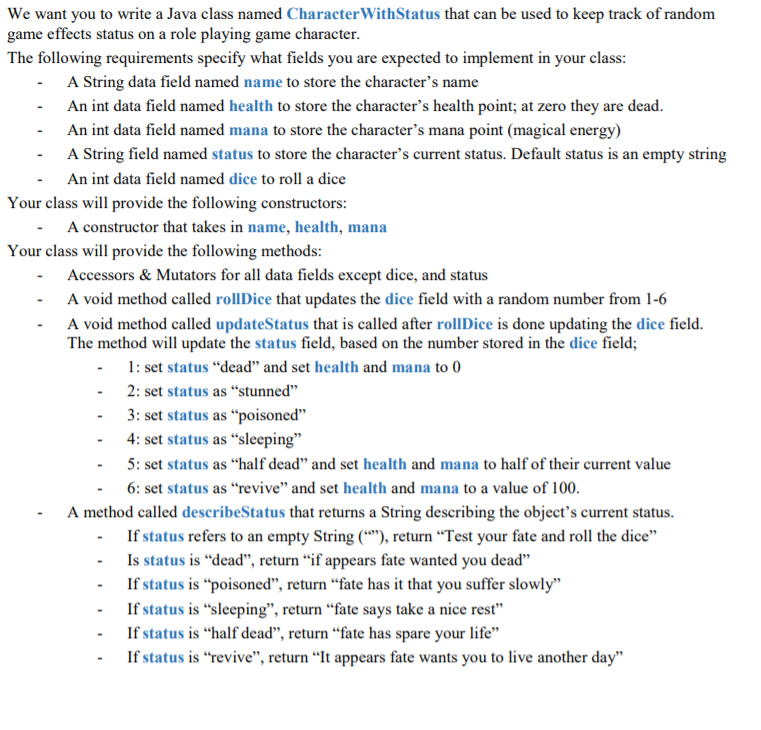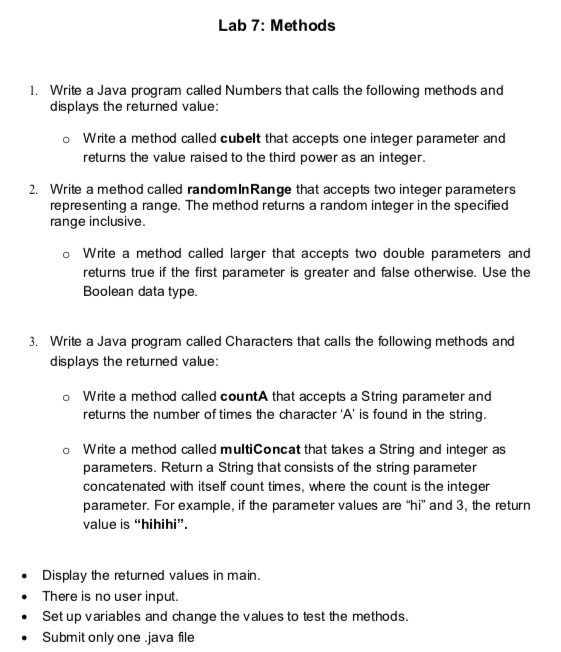Question

# WRITING METHODS 1. Implement a method named surface that accepts 3 integer parameters named width, length,...

WRITING METHODS

1. Implement a method named surface that accepts 3 integer parameters named width, length, and depth. It will return the total surface area (6 sides) of the rectangular box it represents.

2. Implement a method named rightTriangle that accepts 2 double arameters named sideA and hypotenuseB. The method will return the length of the third side.

NOTE
To test, you should put all of these methods into a ‘MyMethods’ class and then write an application that will instantiate an instance (object) of that class to use the above methods. It should be obvious that you will need to get the parameters from either the user or through some other means (random number generator) so that when you call (invoke) the method you have the actual parameters to pass along to the method being called. If you do generate numbers have the application print them out so that you know what was generated and given to the method so that the return values can be checked for correctness.

3. Modify the class Die presented in class to include another instance data (String), called color, to represent the color of a die. Add a getter/setter for this data.

4. Implement a method comboDie that takes two dice parameters. The method returns a die with color the combination of both dice’ colors and face value the integer average of the dice’ facevalues. (Add this method to your MyMethods class)

Example: if first die is “blue” with facevalue=3 and second die is “red”with facevalue=5, the method returns a “blue-red” die with facevalue=4. Note.

Use the Die class presented in class.

DEFINING CLASSES

5. Using the Die class defined in class, design and implement a class called PairOfDice, composed of two Die objects. Include methods to set and get each individual die, a method to roll the dice, a toString method that returns colors of both dice and a method pairSum that returns the current sum of the two die values.

6. Write an application TestPairOfDice that uses the PairOfDice class to create and roll a pair of dice. The application prints to the screen the sum of their fair values.

```public class MyMethods {

public double surface(int width, int length, int depth) {
return 2 * (width * length + width * depth + length * depth);
}

public double rightTriangle(double sideA, double hypotenuseB) {
return Math.sqrt((hypotenuseB * hypotenuseB) - (sideA * sideA));
}
}```

###########################################################################################

```import java.util.Random;

public class MyMethodsTest {

public static void main(String[] args) {
Random random = new Random();

MyMethods methods = new MyMethods();
int length = random.nextInt(20) + 20;
int width = random.nextInt(15) + 8;
int depth = random.nextInt(10) + 5;

System.out.println("Length: " + length + " Width: " + width +
" Depth: " + depth + " Surface Area: " + methods.surface(width, length, depth));

int hypotenuse = random.nextInt(20) + 20;
int sideA = random.nextInt(10) + 5;
System.out.println("Hypotenuse: " + hypotenuse + "\nSide A: " + sideA +
"\nSide B: " + methods.rightTriangle(sideA, hypotenuse));

}
}```

############################################################################################### Earn Coins

Coins can be redeemed for fabulous gifts.

Similar Homework Help Questions
• ### I need help with these Java programming assignements. public class Die { //here you declare your...

I need help with these Java programming assignements. public class Die { //here you declare your attributes private int faceValue; //operations //constructor - public Die() { //body of constructor faceValue=(int)(Math.random()*6)+1;//instead of 1, use random approach to generate it } //roll operation public void roll() { faceValue=(int)(Math.random()*6)+1; }    //add a getter method public int getFaceValue() { return faceValue; }    //add a setter method public void setFaceValue(int value) { faceValue=value; } //add a toString() method public String toString() { String...

• ### Using the Die class defined in this chapter, design and implement a class called PairOfDice, composed...

Using the Die class defined in this chapter, design and implement a class called PairOfDice, composed of two Die objects. Include methods to set and get the individual die values, a method to roll the dice, and a method that returns the current sum of the two die values. Create a driver class called RollingDice2 to instantiate and use a PairOfDice object. I need the main method or test class public class Die { private final int MAX = 6;...

• ### Hello, Could you please help me code this program in Java? It is important that all...

Hello, Could you please help me code this program in Java? It is important that all rules are carefully followed. Using the PairOfDice class from PP 4.9, design and implement a class to play a game called Pig. In this game, the user competes against the computer. On each turn, the current player rolls a pair of dice and accumulates points. The goal is to reach 100 points before your opponent does. If, on any turn, the player rolls a...

• ### Make a public class called ManyExamples and in the class... Write a function named isEven which...

Make a public class called ManyExamples and in the class... Write a function named isEven which takes in a single int parameter and returns a boolean. This function should return true if the given int parameter is an even number and false if the parameter is odd. Write a function named close10 which takes two int parameters and returns an int. This function should return whichever parameter value is nearest to the value 10. It should return 0 in the...

• ### Create 3 user-defined methods called add(). 1 accepts a single integer array parameter; this method should...

Create 3 user-defined methods called add(). 1 accepts a single integer array parameter; this method should loop the array adding the values and return the summation (use 4, 1, 4, 6, 10, 15, 32, 79, 18 as the values in the array) 1 accepts 2 integer parameters; this method should add them and return the value 1 accepts 3 integer parameters; this method should add them all and return the value In main, define the parameters above (you may use...

• ### 3. Write Java methods to accomplish each of the following Write a method named simpleAry which...3. Write Java methods to accomplish each of the following Write a method named simpleAry which accepts and return nothing. It creates an array that can hold ten integers. Fill up each slot of the array with the number 113. Then, display the contents of the array on the screen. You must use a loop to put the values in the array and also to display them. Write a method named sury which accepts an array of type double with...

• ### Create an abstract class “Appointment” that represents an event on a calendar. The “Appointment” class will...

Create an abstract class “Appointment” that represents an event on a calendar. The “Appointment” class will have four instance variables: • An instance variable called “year” which will be of type int • An instance variable called “month” which will be of type int • An instance variable called “day” which will be of type int • An instance variable called “description” which will be of type String The “Appointment” class must also implement the following methods: • A getter...

• ### To be written in JAVA We want you to write a Java class named Character WithStatus...To be written in JAVA We want you to write a Java class named Character WithStatus that can be used to keep track of random game effects status on a role playing game character. The following requirements specify what fields you are expected to implement in your class: - A String data field named name to store the character's name - An int data field named health to store the character's health point; at zero they are dead. - An...

• ### The first programming project involves writing a program that computes the minimum, the maximum and the...

The first programming project involves writing a program that computes the minimum, the maximum and the average weight of a collection of weights represented in pounds and ounces that are read from an input file. This program consists of two classes. The first class is the Weight class, which is specified in integer pounds and ounces stored as a double precision floating point number. It should have five public methods and two private methods: 1. A public constructor that allows...

• ### I need java code for the following problem. Lab 7: Methods 1. Write a Java program called Numbers that calls the following methods and displays the returned value: Write a method called cubelt that a...I need java code for the following problem. Lab 7: Methods 1. Write a Java program called Numbers that calls the following methods and displays the returned value: Write a method called cubelt that accepts one integer parameter and returns the value raised to the third power as an integer. o Write a method called randominRange that accepts two integer parameters representing a range. The method returns a random integer in the specified range inclusive. 2. o Write a method...